SBOS789C August   2017  – February 2020

PRODUCTION DATA.

1. Features
2. Applications
3. Description
1.     Device Images
4. Revision History
5. Device Comparison Table
6. Pin Configuration and Functions
7. Specifications
8. Detailed Description
1. 8.1 Overview
2. 8.2 Functional Block Diagram
3. 8.3 Feature Description
4. 8.4 Device Functional Modes
9. Application and Implementation
1. 9.1 Application Information
2. 9.2 Typical Applications
1. 9.2.1 Transimpedance Amplifier
2. 9.2.2 Multichannel Sensor Interface
10. 10Power Supply Recommendations
11. 11Layout
1. 11.1 Layout Guidelines
2. 11.2 Layout Example
12. 12Device and Documentation Support
13. 13Mechanical, Packaging, and Orderable Information

• D|8
• DCN|8
• DGK|8
• DCN|8
• DGK|8

### 7.8 Typical Characteristics: VS = 10 V

at VS+ = 5 V, VS– = –5 V, RL = 1 kΩ, input and output are biased to midsupply, and TA ≈ 25°C. For AC specifications, VO = 2 VPP, G = 2 V/V, RF = 1 kΩ, and CL = 4.7 pF (unless otherwise noted)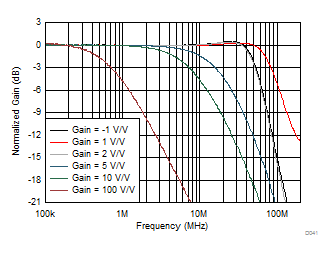See Figure 64 and Figure 65, VO = 20 mVPP
Figure 1. Small-Signal Frequency Response vs Gain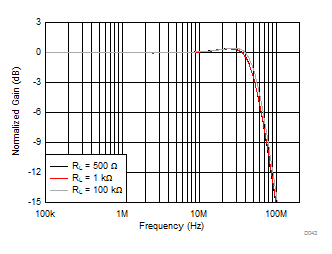See Figure 64, VO = 20 mVPP, Gain = 2 V/V
Figure 3. Small-Signal Frequency Response vs Output Load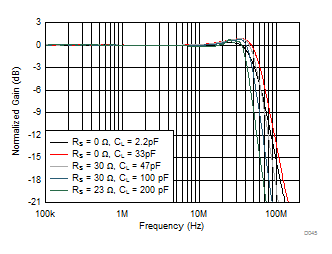See Figure 64and Figure 62, VO = 20 mVPP, Gain = 2 V/V
Figure 5. Small-Signal Frequency Response vs CL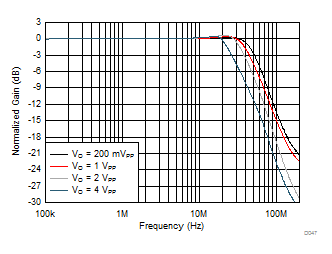See Figure 64, Gain = 2 V/V
Figure 7. Large-Signal Frequency Response vs Output Voltage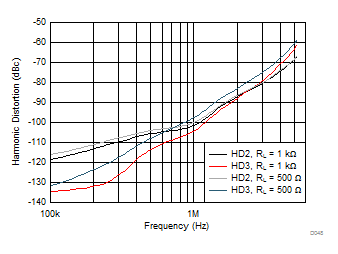See Figure 64, Gain = 2 V/V
Figure 9. Harmonic Distortion vs Frequency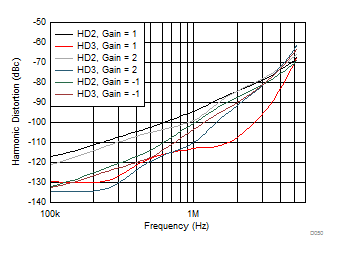See Figure 64 and Figure 65, RF = 0 Ω for Gain = 1 V/V
Figure 11. Harmonic Distortion vs Gain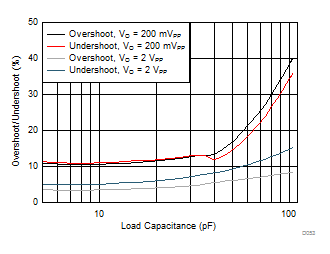See Figure 64, Gain = 1 V/V, RF = 0 Ω
Figure 13. Overshoot and Undershoot vs CL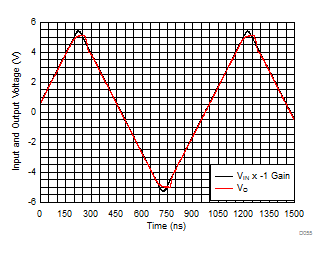See Figure 65, Gain = -1 V/V
Figure 15. Output Overdrive Recovery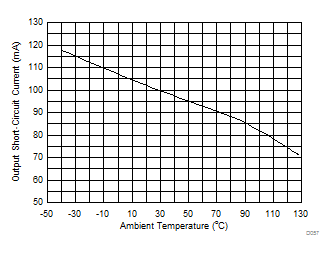Output saturated and then short-circuited, Io measured after TDelay = 5 msec
Figure 17. Output Short-Circuit Current vs Ambient Temperature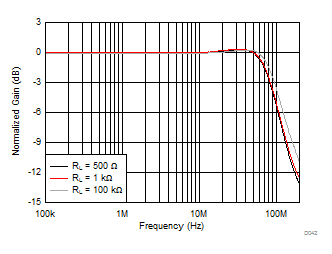See Figure 64, VO = 20 mVPP, Gain = 1 V/V, RF = 0 Ω
Figure 2. Small-Signal Frequency Response vs Output Load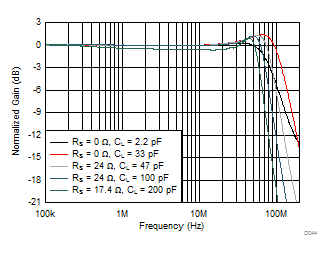See Figure 64 and Figure 62, VO = 20 mVPP, Gain = 1 V/V, RF = 0 Ω
Figure 4. Small-Signal Frequency Response vs CL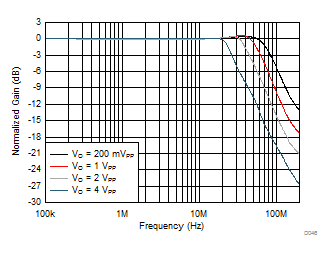See Figure 64, Gain = 1 V/V, RF = 0 Ω
Figure 6. Large-Signal Frequency Response vs Output Voltage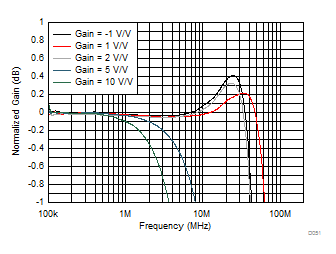See Figure 64 and Figure 65, VO = 20 mVPP
Figure 8. Small-Signal Response Flatness vs Gain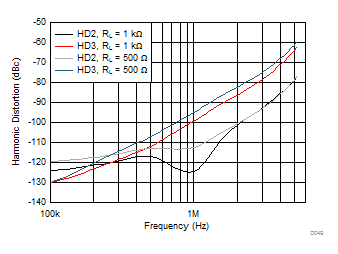See Figure 65, Gain = –1 V/V
Figure 10. Harmonic Distortion vs Frequency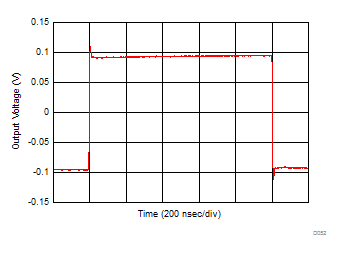See Figure 64, Gain = 1 V/V, RF = 0 Ω
Figure 12. Small-Signal Transient Response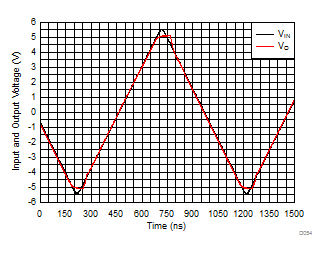See Figure 64, Gain = 1 V/V, RF = 0 Ω
Figure 14. Input Overdrive Recovery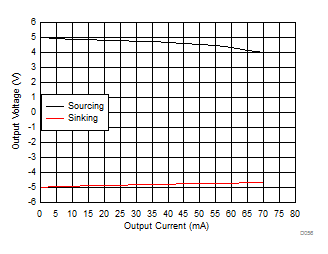Figure 16. Output Voltage vs Load Current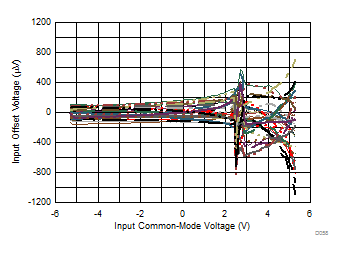Measured for 34 units
Figure 18. Input Offset Voltage vs Input Common-Mode Voltage